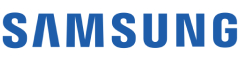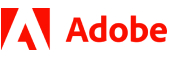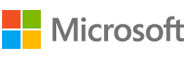New update is available. Click here to update.
Topics

# Reverse Level Order Traversal

Moderate0/80
Average time to solve is 30m+10 more companies

## Problem statement

You have been given a Binary Tree of integers. You are supposed to return the reverse of the level order traversal.

For example:
``````For the given binary tree
````````````The reverse level order traversal will be {7,6,5,4,3,2,1}.
``````
Detailed explanation ( Input/output format, Notes, Images )
Constraints:
``````0 <= N <= 5 * 10^5
0 <= data <= 10^5 and data != -1

Where ‘N’ is the total number of nodes in the binary tree, and 'data' is the value of the binary tree node.

Time Limit: 1 sec
``````
Sample Input 1:
``````1 2 3 4 -1 5 6 -1 7 -1 -1 -1 -1 -1 -1
``````
Sample Output 1:
``````7 6 5 4 3 2 1
``````
Explanation of Sample Input 1:
``````Start with the bottom of the tree, i.e. 7, and traverse in the tree in the reverse direction of level order, i.e., bottom to top and right to left. So, the reverse level order traversal will be {7,6,5,4,3,2,1}.
``````
Sample Input 2:
``````2 7 5 2 6 -1 9 -1 -1 5 11 4 -1 -1 -1 -1 -1 -1 -1
``````
Sample Output 2:
``````4 11 5 9 6 2 5 7 2
``````
Explanation of Sample Input 2:
``````Traverse the tree in the reverse direction of level order, i.e., bottom to top and right to left. So reverse level order traversal will be {4,11,5,9,6,2,5,7,2}.
``````Console